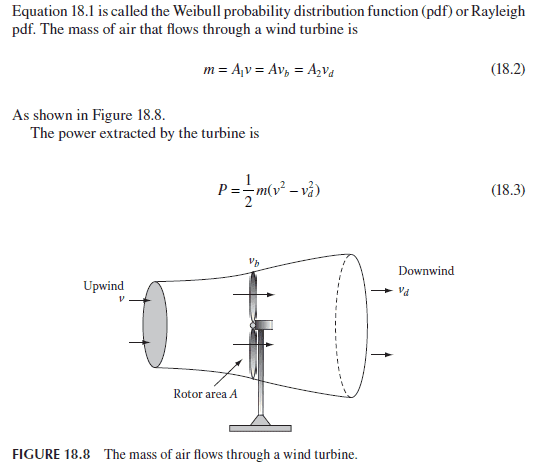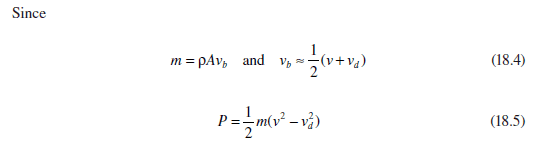# Wind Turbine Power Calculation Equations

Glad it's Friday? I will be if you can help me with this :D :

I'm trying to understand an equation about calculating the power extracted by the blades of a wind turbine. I have spent a couple of hours going over it but still not making much more sense.

I'm trying to find out why P = 1/2*m*(v2 - v2d). Why the 1/2? Why the '-' sign?? And why the 2 ??? I assume it is to do with F=ma, so the v (velocity) is squared to show acceleration but still I can't figure it all out.Any tips on how they got to the last equation (18.5) shown above would be amazing!

Thank you! Cannot wait for this to make sense

•